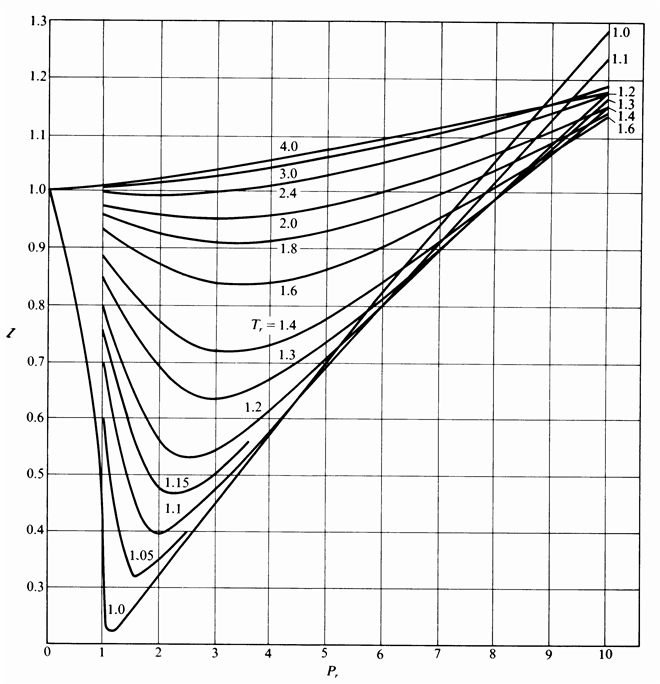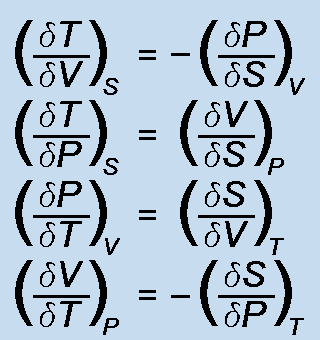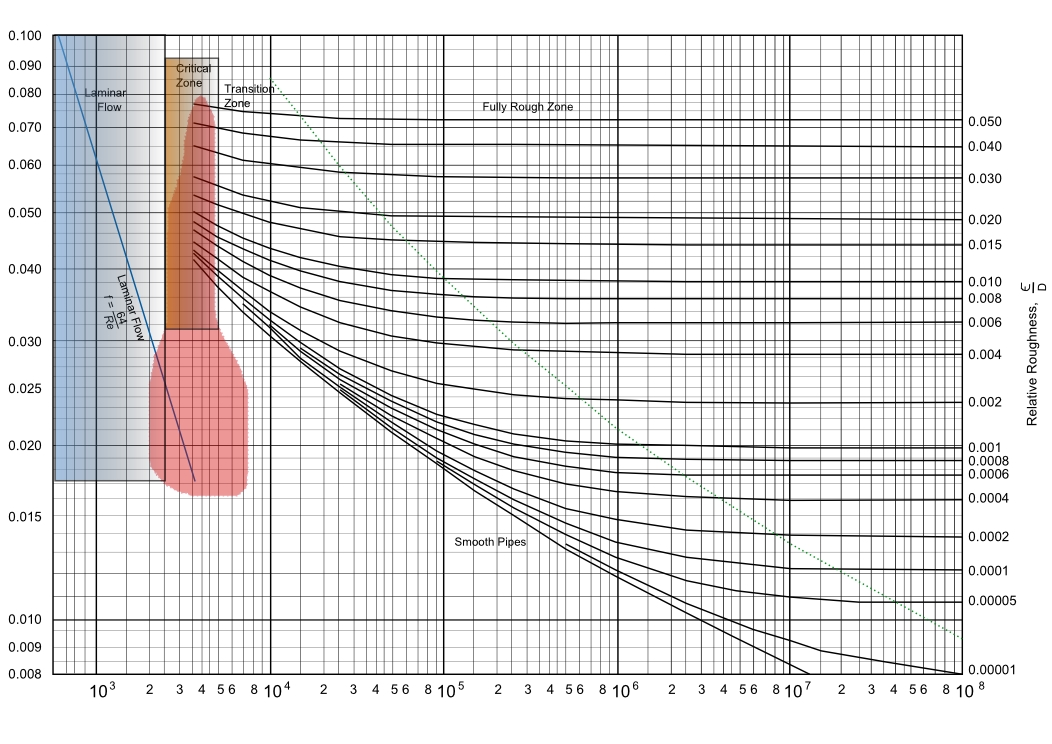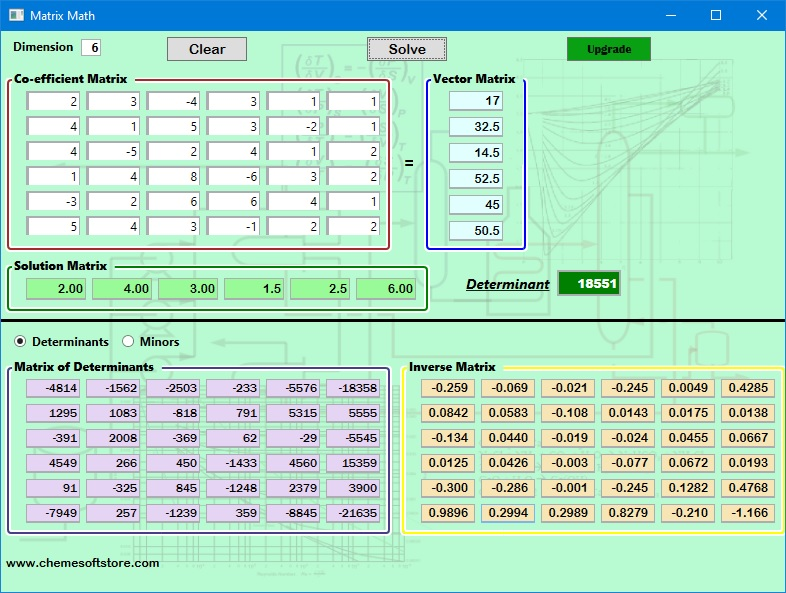### ChemE Soft StoreCaCO3 -> CaO + CO2
NaCl + NH3 + CO2 + H2O -> NaHCO3 + NH4Cl
2 NaHCO3 -> Na2 CO3 + H2O + CO2
CaO + H2 O -> Ca(OH)2
Ca(OH)2 + 2 NH4 Cl -> CaCl2 + 2 NH3 + 2 H2O

## Matrix Math Solver

Solve linear equations up to 6x6 matrices. Results show the solution and inverse matrices, the determinant, as well as the matrices of determinants and minors.#### Features:

• Inverse Matrix calculated and shown
• Both Matrix of Minors and Matrix of Determinants calculated and shown
• Determinant of matrix calculated for all 2 x 2 matrices and greater